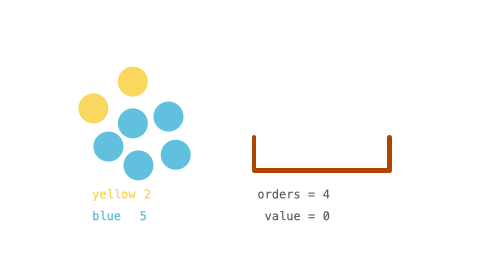# GeetCode Hub

You have an `inventory` of different colored balls, and there is a customer that wants `orders` balls of any color.

The customer weirdly values the colored balls. Each colored ball's value is the number of balls of that color you currently have in your `inventory`. For example, if you own `6` yellow balls, the customer would pay `6` for the first yellow ball. After the transaction, there are only `5` yellow balls left, so the next yellow ball is then valued at `5` (i.e., the value of the balls decreases as you sell more to the customer).

You are given an integer array, `inventory`, where `inventory[i]` represents the number of balls of the `ith` color that you initially own. You are also given an integer `orders`, which represents the total number of balls that the customer wants. You can sell the balls in any order.

Return the maximum total value that you can attain after selling `orders` colored balls. As the answer may be too large, return it modulo `109 + 7`.

Example 1:```Input: inventory = [2,5], orders = 4
Output: 14
Explanation: Sell the 1st color 1 time (2) and the 2nd color 3 times (5 + 4 + 3).
The maximum total value is 2 + 5 + 4 + 3 = 14.
```

Example 2:

```Input: inventory = [3,5], orders = 6
Output: 19
Explanation: Sell the 1st color 2 times (3 + 2) and the 2nd color 4 times (5 + 4 + 3 + 2).
The maximum total value is 3 + 2 + 5 + 4 + 3 + 2 = 19.
```

Example 3:

```Input: inventory = [2,8,4,10,6], orders = 20
Output: 110
```

Example 4:

```Input: inventory = , orders = 1000000000
Output: 21
Explanation: Sell the 1st color 1000000000 times for a total value of 500000000500000000. 500000000500000000 modulo 109 + 7 = 21.
```

Constraints:

• `1 <= inventory.length <= 105`
• `1 <= inventory[i] <= 109`
• `1 <= orders <= min(sum(inventory[i]), 109)`

class Solution { public int maxProfit(int[] inventory, int orders) { } }